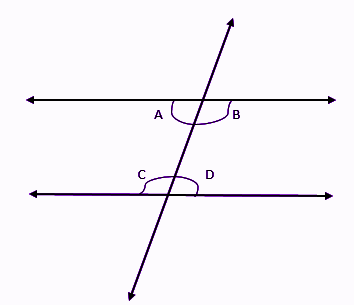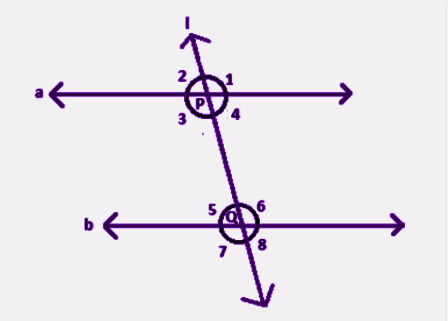# Alternate Interior Angles definitionAlternate Interior Angles: An angle is formed when two rays, a line with one endpoint, meet at one point called a vertex. The angle is formed by the distance between the two rays. Angles in geometry are often referred to using the angle symbol so angle A would be written as angle A.

A straight angle, also called a flat angle, is formed by a straight line. The measure of this angle is 180 degrees. A straight angle can also be formed by two or more angles that sum to 180 degrees. Here, angle 1 + angle 2 = 180.A transversal line is a line that crosses or passes through two other lines. Sometimes, the two other lines are parallel, and the transversal passes through both lines at the same angle. The two other lines don’t necessarily have to be parallel in order for a transversal to cross them.

Alternate Interior Angles interior angles are formed when a transversal passes through two lines. The angle that is formed on opposite sides of the transversal and inside the two lines are alternate interior angle. Notice the pairs of blue and pink angles. Parallel lines are two lines on a two-dimensional plane that never meet or cross. When a transversal passes through parallel lines, there are special properties about the angles that are formed that do not occur when the lines are not parallel. Notice the arrows on lines m and n towards the left. These arrows indicate that lines m and n are parallel.

## Alternate Interior Angles Definition

The angles which are formed inside the two parallel lines, when intersected by a transversal, are equal to its alternate pairs.In the above-given figure, you can see, two parallel lines are intersected by a transversal. Therefore, the alternate angles inside the parallel lines will be equal.

i,e. ∠A = ∠D and ∠B = ∠C

## What Are Alternate Interior Angles/Converse Of  Interior Angles Theorem

The theorem states that “ if a transversal crosses the set of parallel lines, the alternate interior angles are congruent”.

Given: a//b

To prove: ∠4 = ∠5 and ∠3 = ∠6

Proof: Suppose a and b are two parallel lines and l is the transversal which intersects a and b at points P and Q. See the figure.From the properties of the parallel line, we know if a transversal cuts any two parallel lines, the corresponding angles and vertically opposite angles are equal to each other. Therefore,

∠2 = ∠5 ………..(i) [Corresponding angles]

∠2 = ∠4 ………..(ii) [Vertically opposite angles]

From eq.(i) and (ii), we get;

∠4 = ∠5 [Alternate interior angles]

Similarly,

∠3 = ∠6

Hence, it is proved.

### Antithesis of Theorem

If the interior angles produced by the transversal line on two coplanar are congruent, then the two lines are parallel to each other.

Given: ∠4 = ∠5 and ∠3 = ∠6

To prove: a//b

Proof: Since ∠2 = ∠4 [Vertically opposite angles]

So, we can write,

∠2 = ∠5, which are corresponding angles.

Therefore, a is parallel to b.

Alternate interior angles are congruent. Formally, these are two interior angles which lie on different parallel lines and on opposite sides of a

The alternate segment theorem (also known as the tangent-chord theorem) states that in any circle, the angle between a chord and a tangent through one of the endpoints of the chord is equal to the angle in the alternate segment. In the above diagram, the angles of the same color are equal to each other.

. Parallel lines cut. by a transversal.

Alternate interior angles are formed when a transversal passes through two lines. The angles that are formed on opposite sides of the transversal and inside the two lines are alternate interior angles. The theorem says that when the lines are parallel, the alternate interior angles are equal.

Read Also: Antigone Summary

The alternate segment theorem (also known as the tangent-chord theorem) states that in any circle, the angle between a chord and a tangent through one of the endpoints of the chord is equal to the angle in the alternate segment. In the above diagram, the angles of the same color are equal to each other.

## Congruent Alternate Interior Angles

The  Interior Angles theorem states, if two parallel lines are cut by a transversal, then the pairs of alternate interior angles are congruent.

theorem is a proven statement or an accepted idea that has been shown to be true. The converse of this theorem, which is basically the opposite, is also a proven statement: if two lines are cut by a transversal and the alternate interior angles are congruent, then the lines are parallel.

These theorems can be used to solve problems in geometry and to find missing information. This diagram shows which pairs are equal and alternate interior. Notice that the lines are parallel.